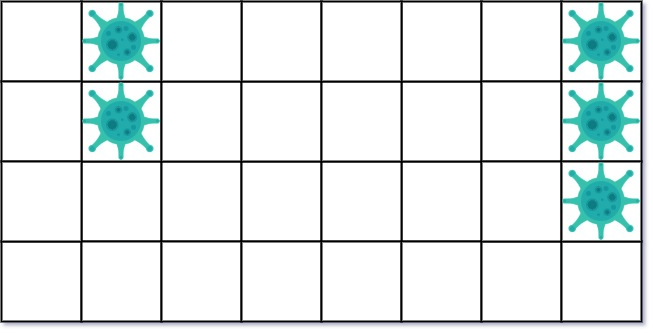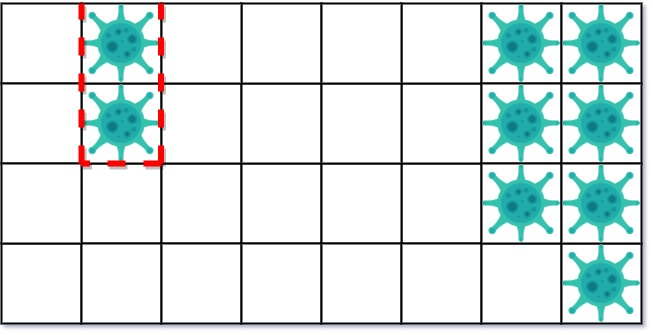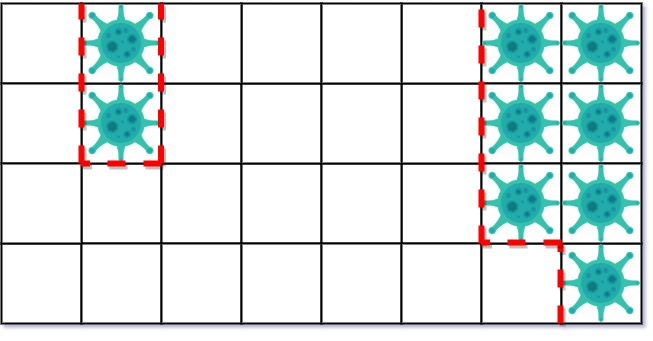## Algorithm

Problem Name: 749. Contain Virus

A virus is spreading rapidly, and your task is to quarantine the infected area by installing walls.

The world is modeled as an `m x n` binary grid `isInfected`, where `isInfected[i][j] == 0` represents uninfected cells, and `isInfected[i][j] == 1` represents cells contaminated with the virus. A wall (and only one wall) can be installed between any two 4-directionally adjacent cells, on the shared boundary.

Every night, the virus spreads to all neighboring cells in all four directions unless blocked by a wall. Resources are limited. Each day, you can install walls around only one region (i.e., the affected area (continuous block of infected cells) that threatens the most uninfected cells the following night). There will never be a tie.

Return the number of walls used to quarantine all the infected regions. If the world will become fully infected, return the number of walls used.

Example 1:```Input: isInfected = [[0,1,0,0,0,0,0,1],[0,1,0,0,0,0,0,1],[0,0,0,0,0,0,0,1],[0,0,0,0,0,0,0,0]]
Output: 10
Explanation: There are 2 contaminated regions.
On the first day, add 5 walls to quarantine the viral region on the left. The board after the virus spreads is:On the second day, add 5 walls to quarantine the viral region on the right. The virus is fully contained.```

Example 2:```Input: isInfected = [[1,1,1],[1,0,1],[1,1,1]]
Output: 4
Explanation: Even though there is only one cell saved, there are 4 walls built.
Notice that walls are only built on the shared boundary of two different cells.
```

Example 3:

```Input: isInfected = [[1,1,1,0,0,0,0,0,0],[1,0,1,0,1,1,1,1,1],[1,1,1,0,0,0,0,0,0]]
Output: 13
Explanation: The region on the left only builds two new walls.
```

Constraints:

• `m == isInfected.length`
• `n == isInfected[i].length`
• `1 <= m, n <= 50`
• `isInfected[i][j]` is either `0` or `1`.
• There is always a contiguous viral region throughout the described process that will infect strictly more uncontaminated squares in the next round.

## Code Examples

### #1 Code Example with Javascript Programming

```Code - Javascript Programming```

``````
const containVirus = function (grid) {
let ans = 0
while (true) {
const walls = model(grid)
if (walls === 0) break
ans += walls
}
return ans
function model(grid) {
const m = grid.length,
n = grid.length
const virus = [],
toInfect = []
const visited = Array.from({ length: m }, () => Array(n).fill(0))
const walls = []
for (let i = 0; i < m; i++) {
for (let j = 0; j < n; j++) {
if (grid[i][j] === 1 && visited[i][j] === 0) {
virus.push(new Set())
toInfect.push(new Set())
walls.push()
dfs(
grid,
visited,
virus[virus.length - 1],
toInfect[toInfect.length - 1],
walls[walls.length - 1],
i,
j
)
}
}
}
let maxArea = 0,
idx = -1
for (let i = 0; i < toInfect.length; i++) {
if (toInfect[i].size > maxArea) {
maxArea = toInfect[i].size
idx = i
}
}
if (idx === -1) return 0
for (let i = 0; i < toInfect.length; i++) {
if (i !== idx) {
for (let key of toInfect[i]) grid[(key / n) >> 0][key % n] = 1
} else {
for (let key of virus[i]) grid[(key / n) >> 0][key % n] = -1
}
}
return walls[idx]
}
function dfs(grid, visited, virus, toInfect, wall, row, col) {
const m = grid.length,
n = grid.length
if (row < 0 || row >= m || col < 0 || col >= n || visited[row][col] === 1)
return
if (grid[row][col] === 1) {
visited[row][col] = 1
const dir = [0, -1, 0, 1, 0]
for (let i = 0; i < 4; i++)
dfs(
grid,
visited,
virus,
toInfect,
wall,
row + dir[i],
col + dir[i + 1]
)
} else if (grid[row][col] === 0) {
wall++
}
}
}
``````
Copy The Code &

Input

cmd
isInfected = [[0,1,0,0,0,0,0,1],[0,1,0,0,0,0,0,1],[0,0,0,0,0,0,0,1],[0,0,0,0,0,0,0,0]]

Output

cmd
10

### #2 Code Example with Python Programming

```Code - Python Programming```

``````
class Solution(object):
def containVirus(self, grid):
"""
:type grid: List[List[int]]
:rtype: int
"""
if not grid: return None
N,M=len(grid),len(grid)

def around(r,c,t=None):
# all cells 1-step away from (r,c)
# optionally, if t!=None, target value must be t
for d in (-1,1):
for (rr,cc) in ((r+d,c), (r,c+d)):
if 0<=rr``````
``` Copy The Code & Input x – + cmd isInfected = [[0,1,0,0,0,0,0,1],[0,1,0,0,0,0,0,1],[0,0,0,0,0,0,0,1],[0,0,0,0,0,0,0,0]] Output x – + cmd 10 ```
``` Demonstration ```
``` #748 Leetcode Shortest Completing Word Solution in C, C++, Java, JavaScript, Python, C# Leetcode #752 Leetcode Open the Lock Solution in C, C++, Java, JavaScript, Python, C# Leetcode ```
``` ```